# 11 Normal Linear Models

## 11.1 Linear Regression

### 11.1.1 Background

Linear regression is the basis of a large part of applied statistical analysis. Analysis of variance (ANOVA) and analysis of covariance (ANCOVA) can be considered special cases of linear regression, and generalized linear models are extensions of linear regression.

Typical questions that can be answered using linear regression are: How does $$y$$ change with changes in $$x$$? How is y predicted from $$x$$? An ordinary linear regression (i.e., one numeric $$x$$ and one numeric $$y$$ variable) can be represented by a scatterplot of $$y$$ against $$x$$. We search for the line that ﬁts best and describe how the observations scatter around this regression line (see Fig. 11.1 for an example). The model formula of a simple linear regression with one continuous predictor variable $$x_i$$ (the subscript $$i$$ denotes the $$i=1,\dots,n$$ data points) is:

\begin{align} \mu_i &=\beta_0 + \beta_1 x_i \\ y_i &\sim Norm(\mu_i, \sigma^2) \tag{11.1} \end{align}

While the first part of Equation (11.1) describes the regression line, the second part describes the differences between predicted values $$\mu_i$$ and observations $$y_i$$. In other words: the observation $$y_i$$ stems from a normal distribution with mean $$\mu_i$$ and variance $$\sigma^2$$ . The mean of the normal distribution, $$\mu_i$$ , equals the sum of the intercept ($$b_0$$ ) and the product of the slope ($$b_1$$) and the continuous predictor value, $$x_i$$.

The differences between observation $$y_i$$ and the predicted values $$\mu_i$$ are the residuals (i.e., $$\epsilon_i=y_i-\mu_i$$). Equivalently to Equation (11.1), the regression could thus be written as:

\begin{align} y_i &= \beta_0 + \beta_1 x_i + \epsilon_i\\ \epsilon_i &\sim Norm(0, \sigma^2) \tag{11.2} \end{align}

We prefer the notation in Equation (11.1) because, in this formula, the stochastic part (second row) is nicely separated from the deterministic part (first row) of the model, whereas, in the second notation (11.2) the ﬁrst row contains both stochastic and deterministic parts.

Using matrix notation equation (11.1) can also be written in one row:

$\boldsymbol{y} \sim Norm(\boldsymbol{X} \boldsymbol{\beta}, \sigma^2\boldsymbol{I})$

where $$\boldsymbol{ I}$$ is the $$n \times n$$ identity matrix (it transforms the variance parameter to a $$n \times n$$ matrix with its diagonal elements equal $$\sigma^2$$ ; $$n$$ is the sample size). The multiplication by $$\boldsymbol{ I}$$ is necessary because we use vector notation, $$\boldsymbol{y}$$ instead of $$y_{i}$$ . Here, $$\boldsymbol{y}$$ is the vector of all observations, whereas $$y_{i}$$ is a single observation, $$i$$. When using vector notation, we can write the linear predictor of the model, $$\beta_0 + \beta_1 x_i$$ , as a multiplication of the vector of the model coefﬁcients

$\boldsymbol{\beta} = \begin{pmatrix} \beta_0 \\ \beta_1 \end{pmatrix}$

times the model matrix

$\boldsymbol{X} = \begin{pmatrix} 1 & x_1 \\ \dots & \dots \\ 1 & x_n \end{pmatrix}$

where $$x_1 , \dots, x_n$$ are the observed values for the predictor variable, $$x$$. The ﬁrst column of $$\boldsymbol{X}$$ contains only ones because the values in this column are multiplied with the intercept, $$\beta_0$$ . To the intercept, the product of the second element of $$\boldsymbol{\beta}$$, $$\beta_1$$ , with each element in the second column of $$\boldsymbol{X}$$ is added to obtain the predicted value for each observation, $$\boldsymbol{\mu}$$:

\begin{align} \boldsymbol{\beta X}= \begin{pmatrix} \beta_0 \\ \beta_1 \end{pmatrix} \times \begin{pmatrix} 1 & x_1 \\ \dots & \dots \\ 1 & x_n \end{pmatrix} = \begin{pmatrix} \beta_0 + \beta_1x_1 \\ \dots \\ \beta_0 + \beta_1x_n \end{pmatrix}= \begin{pmatrix} \hat{y}_1 \\ \dots \\ \hat{y}_n \end{pmatrix} = \boldsymbol{\mu} \tag{11.3} \end{align}

### 11.1.2 Fitting a Linear Regression in R

In Equation (11.1), the predicted (synonym: fitted) values $$\mu_i$$ are directly deﬁned by the model coefﬁcients, $$\beta_{0}$$ and $$\beta_{1}$$ . Therefore, when we can estimate $$\beta_{0}$$, $$\beta_{1}$$ , and $$\sigma^2$$, the model is fully deﬁned . The last parameter $$\sigma^2$$ describes how the observations scatter around the regression line and relies on the assumption that the residuals are normally distributed. The estimates for the model parameters of a linear regression are obtained by searching for the best ﬁtting regression line. To do so, we search for the regression line that minimizes the sum of the squared residuals. This model ﬁtting method is called the least-squares method, abbreviated as LS. It has a very simple solution using matrix algebra (see e.g., Aitkin et al. 2009).

Note that we can apply LS techniques independent of whether we use a Bayesian or frequentist framework to draw inference. In Bayesian statistics, Equation (11.1) is called the data model, because it describes mathematically the process that has (or, better, that we think has) produced the data. This nomenclature also helps to distinguish data models from models for parameters such as prior distributions.

The least-squares estimates for the model parameters of a linear regression are obtained in R using the function lm. For illustration, we ﬁrst simulate a data set and then ﬁt a linear regression to these simulated data. The advantage of simulating data is that the following analyses can be reproduced without having to read data into R.

set.seed(34)            # set a seed for the random number generator
n <- 50                 # sample size
sigma <- 5              # standard deviation of the residuals
b0 <- 2                 # intercept
b1 <- 0.7               # slope

x <- runif(n, 10, 30)   # sample values of the covariate

mu <- b0 + b1 * x
y <- rnorm(n, mu, sd = sigma)

# Save data in table
dat <- tibble(x = x, y = y)

Then, we ﬁt a linear regression to the data to obtain the results of the three parameters of the linear regression, that is intercept, slope and residual standard deviation.

mod <-  lm(y ~ x, data = dat)
coef(mod)
## (Intercept)           x
##   2.0049517   0.6880415
summary(mod)sigma ##  5.04918 The object “mod” produced by lm contains the estimates for the intercept, $$\beta_0$$ , and the slope, $$\beta_1$$. The residual standard deviation $$\sigma^2$$ is extracted using the function summary. We can show the result of the linear regression as a line in a scatter plot with the covariate (x) on the x-axis and the observations (y) on the y-axis (Fig. 11.1).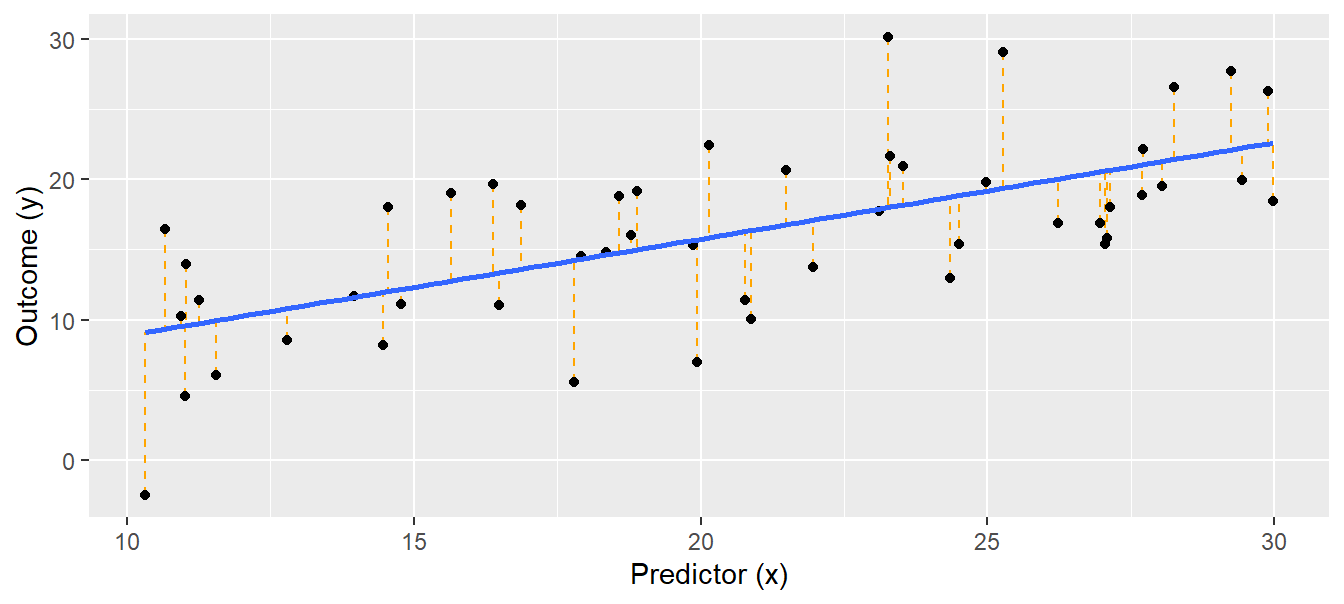Figure 11.1: Linear regression. Black dots = observations, blue solid line = regression line, orange dotted lines = residuals. The ﬁtted values lie where the orange dotted lines touch the blue regression line. Conclusions drawn from a model depend on the model assumptions. When model assumptions are violated, estimates usually are biased and inappropriate conclusions can be drawn. We devote Chapter 12 to the assessment of model assumptions, given its importance. ### 11.1.3 Drawing Conclusions To answer the question about how strongly $$y$$ is related to $$x$$, or to predict $$y$$ from $$x$$, and because we usually draw inference in a Bayesian framework, we are interested in the joint posterior distribution of $$\boldsymbol{\beta}$$ (vector that contains $$\beta_{0}$$ and $$\beta_{1}$$ ) and $$\sigma^2$$ , the residual variance. The function sim does this for us. To somewhat demystify the sim function we brieﬂy explain what sim does. The principle is to ﬁrst draw a random value from the marginal posterior distribution of $$\sigma^2$$, and then to draw random values from the conditional posterior distribution for $$\boldsymbol{\beta}$$ . The conditional posterior distribution of the parameter vector $$\boldsymbol{\beta}$$, $$p(\boldsymbol{\beta}|\sigma^2,\boldsymbol{y,X})$$ is the posterior distribution of $$\boldsymbol{\beta}$$ given a speciﬁc value for $$\sigma^2$$ . This conditional distribution can be analytically derived. With ﬂat prior distributions, it is a uni- or multivariate normal distribution $$p(\boldsymbol{\beta}|\sigma^2,\boldsymbol{y,X})=Norm(\boldsymbol{\hat{\beta}},V_\beta,\sigma^2)$$ with: \begin{align} \boldsymbol{\hat{\beta}=(\boldsymbol{X^TX})^{-1}X^Ty} \tag{11.4} \end{align} and $$V_\beta = (\boldsymbol{X^T X})^{-1}$$ . For models with the normal error distribution, the LS estimates for $$\boldsymbol{\beta}$$ (given by Eq. (11.4)) equal the maximum likelihood (ML) estimates ==(see Chapter 5)==. The marginal posterior distribution of $$\sigma^2$$ is independent of speciﬁc values of $$\boldsymbol{\beta}$$. It is, for ﬂat prior distributions, an inverse chi-square distribution $$p(\sigma^2|\boldsymbol{y,X})=Inv-\chi^2(n-k,\sigma^2)$$, where $$\sigma^2 = \frac{1}{n-k}(\boldsymbol{y}-\boldsymbol{X,\hat{\beta}})^T(\boldsymbol{y}-\boldsymbol{X,\hat{\beta}})$$, and $$k$$ is the number of parameters. The marginal posterior distribution of $$\boldsymbol{\beta}$$ can be obtained by integrating the conditional posterior distribution $$p(\boldsymbol{\beta}|\sigma^2,\boldsymbol{y,X})=Norm(\boldsymbol{\hat{\beta}},V_\beta\sigma^2)$$ over the distribution of $$\sigma^2$$ . This results in a uni- or multivariate $$t$$-distribution. However, it is not necessary to do this analytically. Using the function sim from the package, we can draw samples from $$p(\sigma^2|\boldsymbol{y,X})$$ and describe the marginal posterior distributions of $$\boldsymbol{\beta}$$ using the simulated values. library(arm) nsim <- 1000 bsim <- sim(mod, n.sim = nsim) The function sim simulates (in our example) 1000 values from the joint posterior distribution of the three model parameters $$\beta_0$$ , $$\beta_1$$, and $$\sigma$$. These simulated values are shown in Figure 11.2.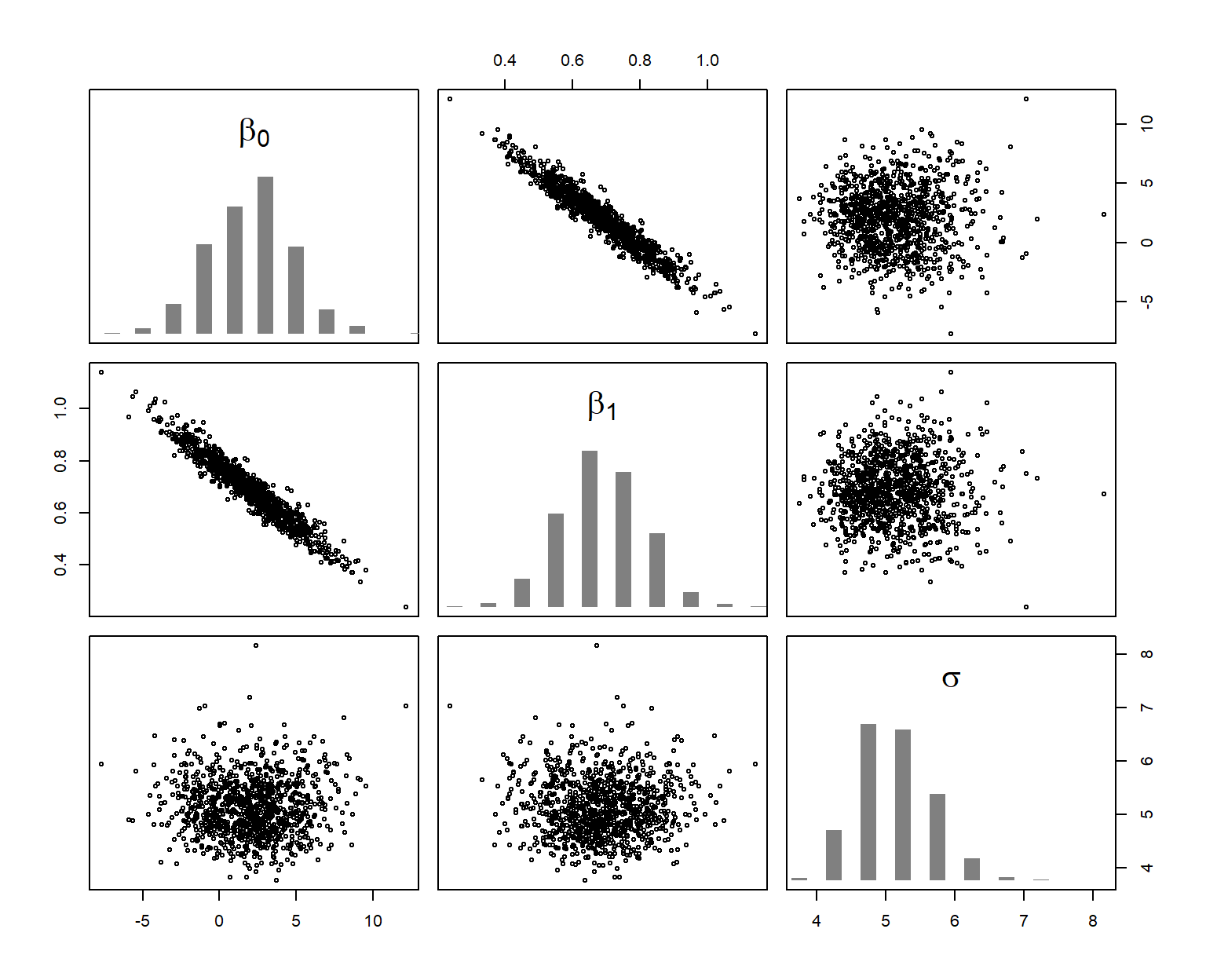Figure 11.2: Joint (scatterplots) and marginal (histograms) posterior distribution of the model parameters. The six scatterplots show, using different axes, the three-dimensional cloud of 1000 simulations from the joint posterior distribution of the three parameters. The posterior distributions describe the range of plausible parameter values given the data and the model. They express our uncertainty about the model parameters; they show what we know about the model parameters after having looked at the data and given the model is realistic. The 2.5% and 97.5% quantiles of the marginal posterior distributions can be used as 95% credible intervals of the model parameters. The function coef extracts the simulated values for the beta coefﬁcients, returning a matrix with nsim rows and the number of columns corresponding to the number of parameters. In our example, the ﬁrst column contains the simulated values from the posterior distribution of the intercept and the second column contains values from the posterior distribution of the slope. The “2” in the second argument of the apply-function (see Chapter ??) indicates that the quantile function is applied columnwise. apply(X = coef(bsim), MARGIN = 2, FUN = quantile, probs = c(0.025, 0.975)) %>% round(2) ## (Intercept) x ## 2.5% -2.95 0.44 ## 97.5% 7.17 0.92 We also can calculate a credible interval of the estimated residual standard deviation, $$\hat{\sigma}$$. quantile(bsim@sigma, probs = c(0.025, 0.975)) %>% round(1) ## 2.5% 97.5% ## 4.2 6.3 Using Bayesian methods allows us to get a posterior probability for speciﬁc hypotheses, such as “The slope parameter is larger than 1” or “The slope parameter is larger than 0.5.” These probabilities are the proportion of simulated values from the posterior distribution that are larger than 1 and 0.5, respectively. sum(coef(bsim)[,2] > 1) / nsim ##  0.008 sum(coef(bsim)[,2] > 0.5) / nsim ##  0.936 From this, there is very little evidence in the data that the slope is larger than 1, but we are quite conﬁdent that the slope is larger than 0.5 (assuming that our model is realistic). We often want to show the effect of $$x$$ on $$y$$ graphically, with information about the uncertainty of the parameter estimates included in the graph. To draw such effect plots, we use the simulated values from the posterior distribution of the model parameters. From the deterministic part of the model, we know the regression line $$\mu = \beta_0 + \beta_1 x_i$$. The simulation from the joint posterior distribution of $$\beta_0$$ and $$\beta_1$$ gives 1000 pairs of intercepts and slopes that describe 1000 different regression lines. We can draw these regression lines in an x-y plot (scatter plot) to show the uncertainty in the regression line estimation (Fig. 11.3, left). Note, that in this case it is not advisable to use ggplot because we draw many lines in one plot, which makes ggplot rather slow. par(mar = c(4, 4, 0, 0)) plot(x, y, pch = 16, las = 1, xlab = "Covariate (x)", ylab = "Dependend variable (y)") for(i in 1:nsim) { abline(coef(bsim)[i,1], coef(bsim)[i,2], col = rgb(0, 0, 0, 0.05)) }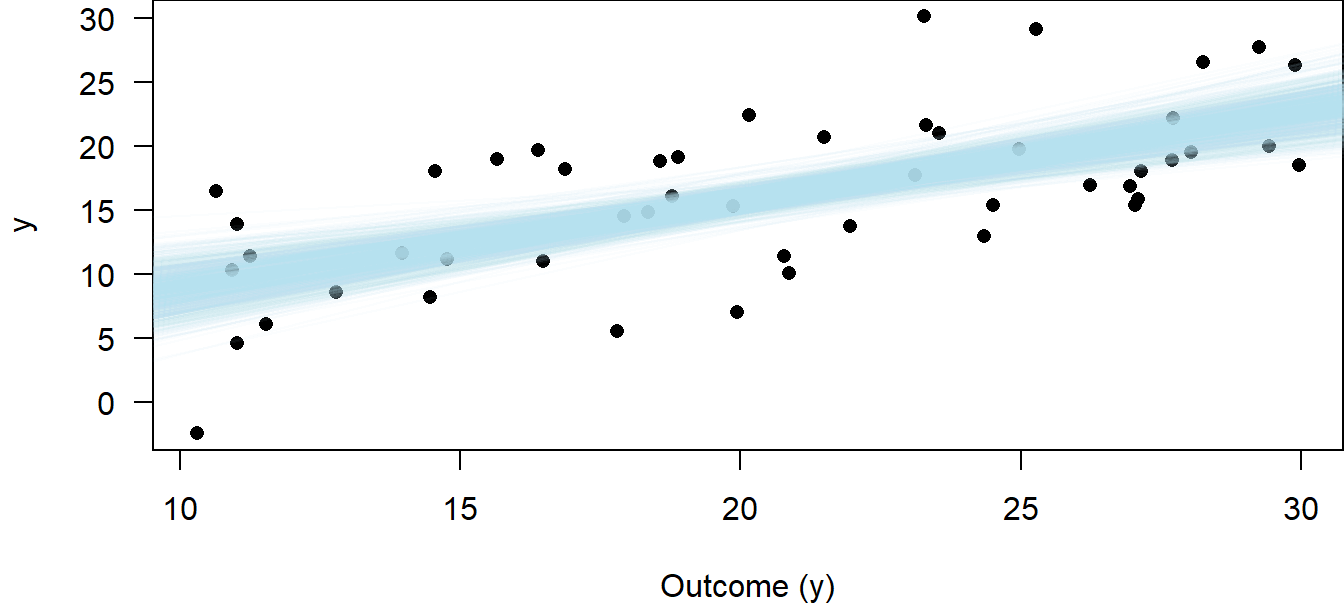Figure 11.3: Regression with 1000 lines based on draws form the joint posterior distribution for the intercept and slope parameters to visualize the uncertainty of the estimated regression line. A more convenient way to show uncertainty is to draw the 95% credible interval, CrI, of the regression line. To this end, we ﬁrst deﬁne new x-values for which we would like to have the ﬁtted values (about 100 points across the range of x will produce smooth-looking lines when connected by line segments). We save these new x-values within the new tibble newdat. Then, we create a new model matrix that contains these new x-values (newmodmat) using the function model.matrix. We then calculate the 1000 ﬁtted values for each element of the new x (one value for each of the 1000 simulated regressions, Fig. 11.3), using matrix multiplication (%*%). We save these values in the matrix “ﬁtmat.” Finally, we extract the 2.5% and 97.5% quantiles for each x-value from ﬁtmat, and draw the lines for the lower and upper limits of the credible interval (Fig. 11.4). # Calculate 95% credible interval newdat <- tibble(x = seq(10, 30, by = 0.1)) newmodmat <- model.matrix( ~ x, data = newdat) fitmat <- matrix(ncol = nsim, nrow = nrow(newdat)) for(i in 1:nsim) {fitmat[,i] <- newmodmat %*% coef(bsim)[i,]} newdatCrI_lo <- apply(fitmat, 1, quantile, probs = 0.025)
newdat$CrI_up <- apply(fitmat, 1, quantile, probs = 0.975) # Make plot regplot <- ggplot(dat, aes(x = x, y = y)) + geom_point() + geom_smooth(method = lm, se = FALSE) + geom_line(data = newdat, aes(x = x, y = CrI_lo), lty = 3) + geom_line(data = newdat, aes(x = x, y = CrI_up), lty = 3) + labs(x = "Covariate (x)", y = "Dependend variable (y)") regplot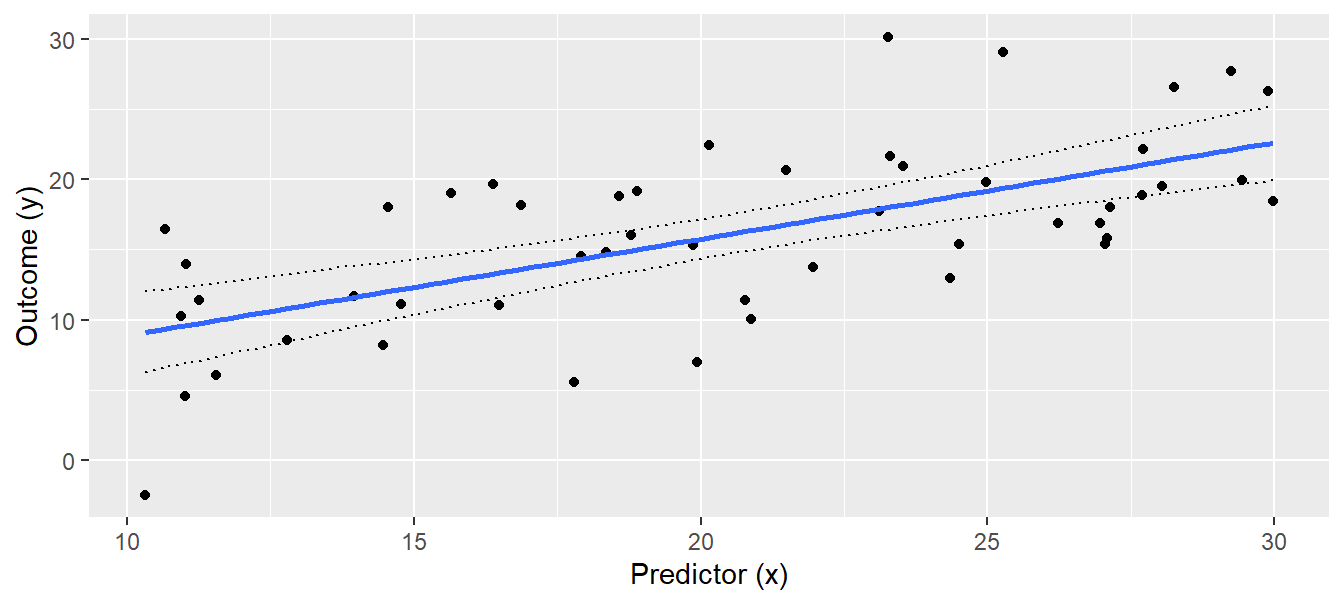Figure 11.4: Regression with 95% credible interval of the posterior distribution of the ﬁtted values. The interpretation of the 95% credible interval is straightforward: We are 95% sure that the true regression line is within the credible interval. As always, this interpretation is only true if the the model is correct. The larger the sample size, the narrower the interval, because each additional data point increases information about the true regression line. The credible interval measures uncertainty of the regression line, but it does not describe how new observations would scatter around the regression line. If we want to describe where future observations will be, we have to report the posterior predictive distribution. We can get a sample of random draws from the posterior predictive distribution $$\hat{y}|\boldsymbol{\beta},\sigma^2,\boldsymbol{X}\sim Norm( \boldsymbol{X \beta, \sigma^2})$$ using the simulated joint posterior distributions of the model parameters, thus taking the uncertainty of the parameter estimates into account. We draw a new $$\hat{y}$$-value from $$Norm( \boldsymbol{X \beta, \sigma^2})$$ for each simulated set of model parameters. Then, we can visualize the 2.5% and 97.5% quantiles of this distribution for each new x-value. # increase number of simulation to procude smooth lines of the posterior # predictive distribution set.seed(34) nsim <- 50000 bsim <- sim(mod, n.sim=nsim) fitmat <- matrix(ncol=nsim, nrow=nrow(newdat)) for(i in 1:nsim) fitmat[,i] <- newmodmat%*%coef(bsim)[i,] # prepare matrix for simulated new data newy <- matrix(ncol=nsim, nrow=nrow(newdat)) # for each simulated ﬁtted value, simulate one new y-value for(i in 1:nsim) { newy[,i] <- rnorm(nrow(newdat), mean = fitmat[,i], sd = bsim@sigma[i]) } # Calculate 2.5% and 97.5% quantiles newdat$pred_lo <- apply(newy, 1, quantile, probs = 0.025)
newdat$pred_up <- apply(newy, 1, quantile, probs = 0.975) # Add the posterior predictive distribution to plot regplot + geom_line(data = newdat, aes(x = x, y = pred_lo), lty = 2) + geom_line(data = newdat, aes(x = x, y = pred_up), lty = 2)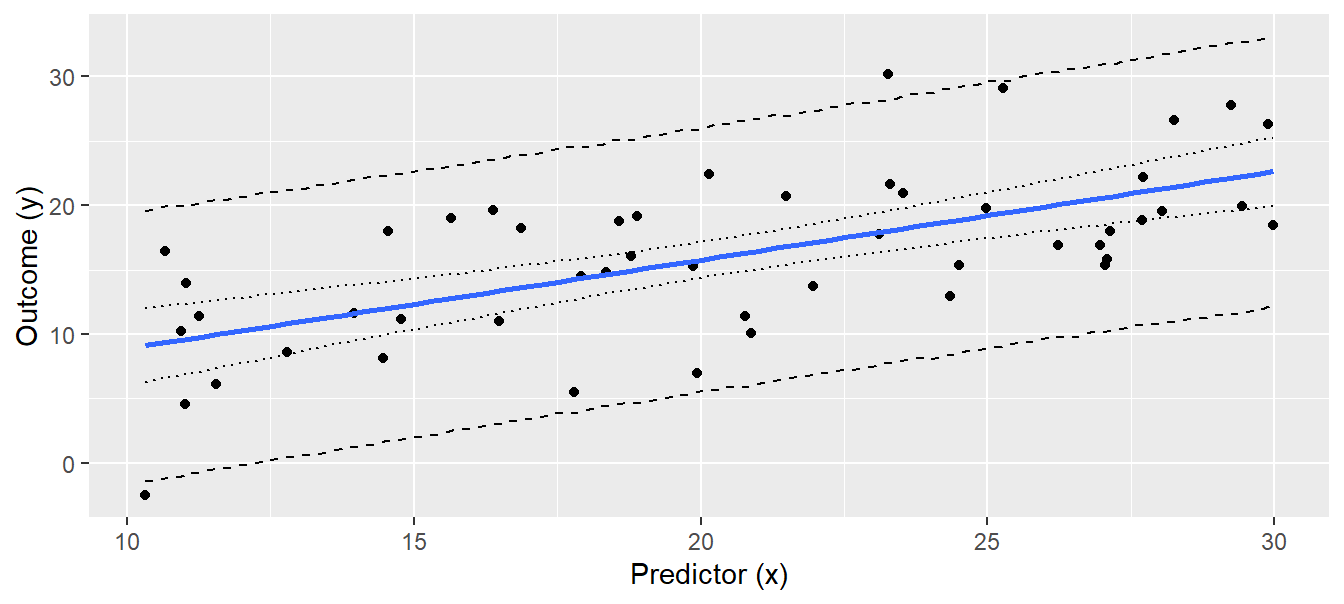Figure 11.5: Regression line with 95% credible interval (dotted lines) and the 95% interval of the simulated predictive distribution (broken lines). Note that we increased the number of simulations to 50,000 to produce smooth lines. Future observations are expected to be within the interval deﬁned by the broken lines in Fig. 11.5 with a probability of 0.95. Increasing sample size will not necessarily give a narrower predictive distribution because the predictive distribution also depends on the residual variance $$\sigma^2$$. The way we produced Fig. 11.5 is somewhat tedious compared to how easy we could have obtained the same ﬁgure using frequentist methods: predict(mod, newdata = newdat, interval = "prediction") would have produced the y-values for the lower and upper lines in Fig. 11.5 in one R-code line. However, once we have a simulated sample of the posterior predictive distribution, we have much more information than is contained in the frequentist prediction interval. For example, we could give an estimate for the proportion of observations greater than 20, given $$x = 25$$. sum(newy[newdat$x == 25, ] > 20) / nsim
##  0.44504

Thus, we expect 44% of future observations with $$x = 25$$ to be higher than 20. We can extract similar information for any relevant threshold value.

Another reason to learn the more complicated R code we presented here, compared to the frequentist methods, is that, for more complicated models such as mixed models, the frequentist methods to obtain conﬁdence intervals of ﬁtted values are much more complicated than the Bayesian method just presented. The latter can be used with only slight adaptations for mixed models and also for generalized linear mixed models.

### 11.1.4 Frequentist Results

The solution for $$\boldsymbol{\beta}$$ is the Equation (11.3). Most statistical software, including R, return an estimated frequentist standard error for each $$\beta_k$$. We extract these standard errors together with the estimates for the model parameters using the summary function.

summary(mod)
##
## Call:
## lm(formula = y ~ x, data = dat)
##
## Residuals:
##      Min       1Q   Median       3Q      Max
## -11.5777  -3.6280  -0.0532   3.9873  12.1374
##
## Coefficients:
##             Estimate Std. Error t value    Pr(>|t|)
## (Intercept)   2.0050     2.5349   0.791       0.433
## x             0.6880     0.1186   5.800 0.000000507 ***
## ---
## Signif. codes:  0 '***' 0.001 '**' 0.01 '*' 0.05 '.' 0.1 ' ' 1
##
## Residual standard error: 5.049 on 48 degrees of freedom
## Multiple R-squared:  0.412,  Adjusted R-squared:  0.3998
## F-statistic: 33.63 on 1 and 48 DF,  p-value: 0.0000005067

The summary output ﬁrst gives a rough summary of the residual distribution. However, we will do more rigorous residual analyses in Chapter 12. The estimates of the model coefﬁcients follow. The column “Estimate” contains the estimates for the intercept $$\beta_0$$ and the slope $$\beta_1$$ . The column “Std. Error” contains the estimated (frequentist) standard errors of the estimates. The last two columns contain the t-value and the p-value of the classical t-test for the null hypothesis that the coefﬁcient equals zero. The last part of the summary output gives the parameter $$\sigma$$ of the model, named “residual standard error” and the residual degrees of freedom.

We try to avoid the name “residual standard error” and use “sigma” instead, because $$\sigma$$ is not a measurement of uncertainty of a parameter estimate like the standard errors of the model coefﬁcients are. $$\sigma$$ is a model parameter that describes how the observations scatter around the ﬁtted values, that is, it is a standard deviation. It is independent of sample size, whereas the standard errors of the estimates for the model parameters will decrease with increasing sample size. Such a standard error of the estimate of $$\sigma$$, however, is not given in the summary output. Note that, by using Bayesian methods, we could easily obtain the standard error of the estimated $$\sigma$$ by calculating the standard deviation of the posterior distribution of $$\sigma$$. The $$R^2$$ and the adjusted $$R^2$$ are explained in Section == “Posterior Predictive Model Checking and Proportion of Explained Variance”==.

## 11.2 Regression Variants: ANOVA, ANCOVA, and Multiple Regression

### 11.2.1 One-Way ANOVA

The aim of analysis of variance (ANOVA) is to compare means of an outcome variable y between different groups (categorical variables). To do so in the frequentist’s framework, variances between and within the groups are compared (hence the name “analysis of variance”). If the variance between the group means is larger than expected by chance , we reject the null hypothesis of no differences between the groups. When doing an ANOVA in a Bayesian way, inference is based on the posterior distributions of the group means and the differences between the group means.

One-way ANOVA means that we only have one explanatory variable (a factor). We illustrate the one-way ANOVA based on an example of simulated data (Fig. 11.6). We have measured weights of 30 virtual individuals for each of 3 groups. Possible research questions could be: How big are the differences between the group means? Are individuals from group 2 heavier than the ones from group 1? Which group mean is higher than 7.5 g?

# settings for the simulation
set.seed(626436)
b0 <- 12        # mean of group 1 (reference group)
sigma <- 2      # residual standard deviation
b1 <- 3         # difference between group 1 and group 2
b2 <- -5        # difference between group 1 and group 3
n <- 90         # sample size

# generate data
group <- factor(rep(c("group 1","group 2", "group 3"), each=30))
simresid <- rnorm(n, mean=0, sd=sigma)  # simulate residuals
y <- b0 +
as.numeric(group=="group 2") * b1 +
as.numeric(group=="group 3") * b2 +
simresid
dat <- tibble(y, group)

# make figure
dat %>%
ggplot(aes(x = group, y = y)) +
geom_boxplot(fill = "orange") +
labs(y = "Weight (g)", x = "") +
ylim(0, NA)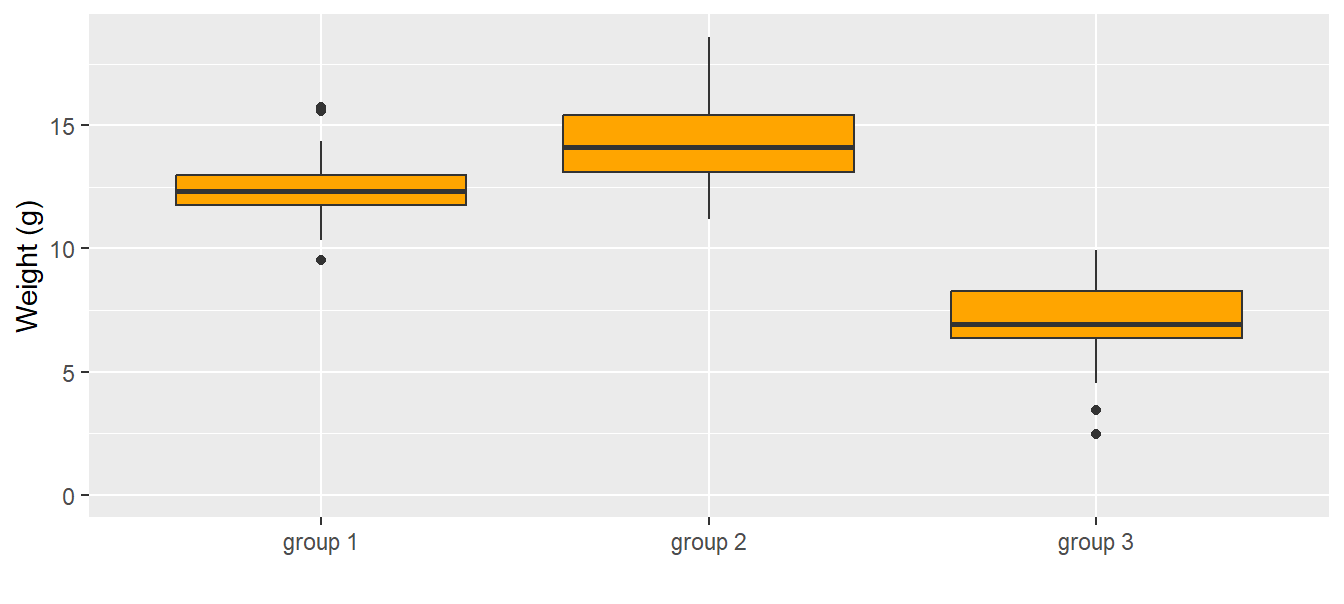Figure 11.6: Weights (g) of the 30 individuals in each group. The dark horizontal line is the median, the box contains 50% of the observations (i.e., the interquartile range), the whiskers mark the range of all observations that are less than 1.5 times the interquartile range away from the edge of the box.

An ANOVA is a linear regression with a categorical predictor variable instead of a continuous one. The categorical predictor variable with $$k$$ levels is (as a default in R) transformed to $$k-1$$ indicator variables. An indicator variable is a binary variable containing 0 and 1 where 1 indicates a speciﬁc level (a category of a nominal variable). Often, one indicator variable is constructed for every level except for the reference level. In our example, the categorical variable is group ($$g$$) with the three levels “group 1,” “group 2,” and “group 3” ($$k = 3$$). Group 1 is taken as the reference level, and for each of the other two groups an indicator variable is constructed, $$I(g_i = 2)$$ and $$I(g_i = 3)$$. We can write the model as a formula:

\begin{align} \mu_i &=\beta_0 + \beta_1 I(g_i=2) + \beta_1 I(g_i=3) \\ y_i &\sim Norm(\mu_i, \sigma^2) \tag{11.5} \end{align}

where $$yi$$ is the $$i$$-th observation (weight measurement for individual i in our example), and $$\beta_{0,1,2}$$ are the model coefﬁcients. The residual variance is $$\sigma^2$$. The model coefﬁcients $$\beta_{0,1,2}$$ constitute the deterministic part of the model. From the model formula it follows that the group means, $$m_g$$, are:

\begin{align} m_1 &=\beta_0 \\ m_2 &=\beta_0 + \beta_1 \\ m_3 &=\beta_0 + \beta_2 \\ \tag{11.6} \end{align}

There are other possibilities to describe three group means with three parameters, for example:

\begin{align} m_1 &=\beta_1 \\ m_2 &=\beta_2 \\ m_3 &=\beta_3 \\ \tag{11.7} \end{align}

In this case, the model formula would be:

\begin{align} \mu_i &= \beta_1 I(g_i=1) + \beta_2 I(g_i=2) + \beta_3 I(g_i=3) \\ y_i &\sim Norm(\mu_i, \sigma^2) \tag{11.8} \end{align}

The way the group means are described is called the parameterization of the model. Different statistical softwares use different parameterizations. The parameterization used by R by default is the one shown in Equation (11.5). R automatically takes the ﬁrst level as the reference (the ﬁrst level is the ﬁrst one alphabetically unless the user deﬁnes a different order for the levels). The mean of the ﬁrst group (i.e., of the ﬁrst factor level) is the intercept, $$b_0$$ , of the model. The mean of another factor level is obtained by adding, to the intercept, the estimate of the corresponding parameter (which is the difference from the reference group mean). R calls this parameterization “treatment contrasts.”

The parameterization of the model is deﬁned by the model matrix. In the case of a one-way ANOVA, there are as many columns in the model matrix as there are factor levels (i.e., groups); thus there are k factor levels and k model coefﬁcients. Recall from Equation (11.3) that for each observation, the entry in the $$j$$-th column of the model matrix is multiplied by the $$j$$-th element of the model coefﬁcients and the $$k$$ products are summed to obtain the ﬁtted values. For a data set with $$n = 5$$ observations of which the ﬁrst two are from group 1, the third from group 2, and the last two from group 3, the model matrix used for the parameterization described in Equation (11.6) is

\begin{align} \boldsymbol{X}= \begin{pmatrix} 1 & 0 & 0 \\ 1 & 0 & 0 \\ 1 & 1 & 0 \\ 1 & 0 & 1 \\ 1 & 0 & 1 \\ \end{pmatrix} \end{align}

If parameterization of Equation (11.7) were used,

\begin{align} \boldsymbol{X}= \begin{pmatrix} 1 & 0 & 0 \\ 1 & 0 & 0 \\ 0 & 1 & 0 \\ 0 & 0 & 1 \\ 0 & 0 & 1 \\ \end{pmatrix} \end{align}

Other possibilities of model parameterizations, particularly for ordered factors, are introduced in Section 11.2.8.

To obtain the parameter estimates for model parameterized according to Equation (11.6) we ﬁt the model in R:

# fit the model
mod <- lm(y~group)

# parameter estimates
mod
##
## Call:
## lm(formula = y ~ group)
##
## Coefficients:
##  (Intercept)  groupgroup 2  groupgroup 3
##       12.367         2.215        -5.430
summary(mod)\$sigma
##  1.684949

The “Intercept” is $$\beta_0$$. The other coefﬁcients are named with the factor name (“group”) and the factor level (either “group 2” or “group 3”). These are $$\beta_1$$ and $$\beta_2$$ , respectively. Before drawing conclusions from an R output we need to examine whether the model assumptions are met, that is, we need to do a residual analysis as described in Chapter 12.

Different questions can be answered using the above ANOVA: What are the group means? What is the difference in the means between group 1 and group 2? What is the difference between the means of the heaviest and lightest group? In a Bayesian framework we can directly assess how strongly the data support the hypothesis that the mean of the group 2 is larger than the mean of group 1. We ﬁrst simulate from the posterior distribution of the model parameters.

library(arm)
nsim <- 1000
bsim <- sim(mod, n.sim=nsim)

Then we obtain the posterior distributions for the group means according to the parameterization of the model formula (Equation (11.6)).

m.g1 <- coef(bsim)[,1]
m.g2 <- coef(bsim)[,1] + coef(bsim)[,2]
m.g3 <- coef(bsim)[,1] + coef(bsim)[,3] 

The histograms of the simulated values from the posterior distributions of the three means are given in Fig. 11.7. The three means are well separated and, based on our data, we are conﬁdent that the group means differ. From these simulated posterior distributions we obtain the means and use the 2.5% and 97.5% quantiles as limits of the 95% credible intervals (Fig. 11.7, right).

# save simulated values from posterior distribution in  tibble
post <-
tibble(group 1 = m.g1, group 2 = m.g2, group 3 = m.g3) %>%
gather("groups", "Group means")

# histograms per group
leftplot <-
ggplot(post, aes(x = Group means, fill = groups)) +
geom_histogram(aes(y=..density..), binwidth = 0.5, col = "black") +
labs(y = "Density") +
theme(legend.position = "top", legend.title = element_blank())

# plot mean and 95%-CrI
rightplot <-
post %>%
group_by(groups) %>%
dplyr::summarise(
mean = mean(Group means),
CrI_lo = quantile(Group means, probs = 0.025),
CrI_up = quantile(Group means, probs = 0.975)) %>%
ggplot(aes(x = groups, y = mean)) +
geom_point() +
geom_errorbar(aes(ymin = CrI_lo, ymax = CrI_up), width = 0.1) +
ylim(0, NA) +
labs(y = "Weight (g)", x ="")

multiplot(leftplot, rightplot, cols = 2)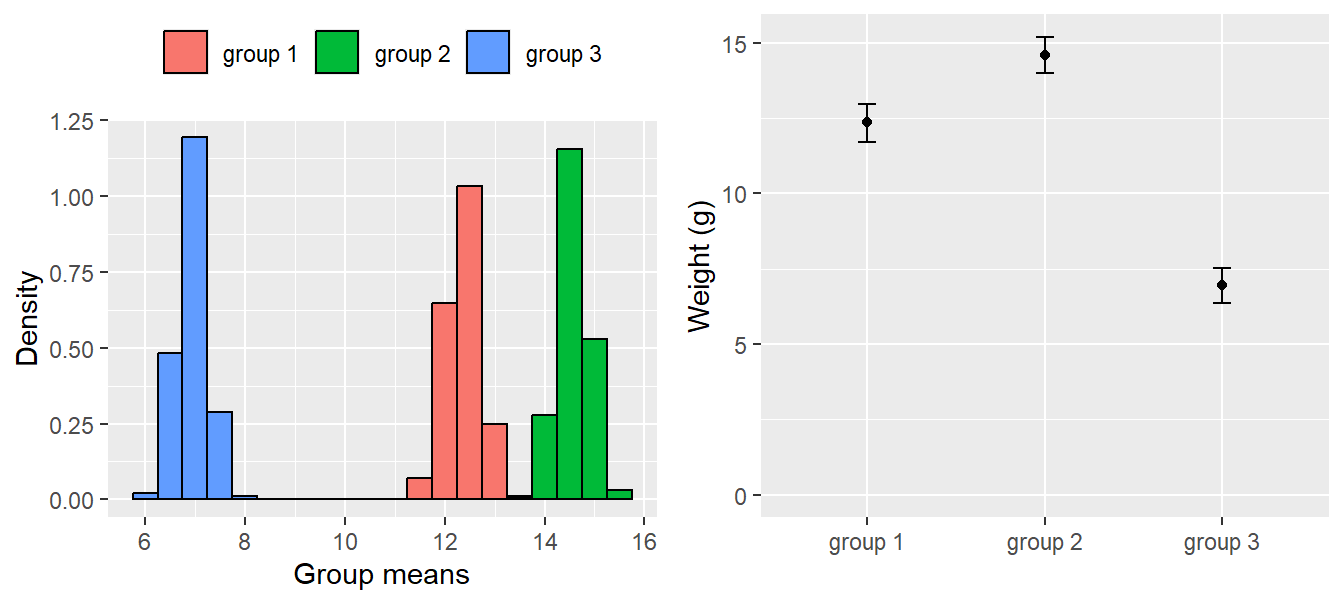Figure 11.7: Distribution of the simulated values from the posterior distributions of the group means (left); group means with 95% credible intervals obtained from the simulated distributions (right).

To obtain the posterior distribution of the difference between the means of group 1 and group 2, we simply calculate this difference for each draw from the joint posterior distribution of the group means.

d.g1.2 <- m.g1 - m.g2
mean(d.g1.2)
##  -2.209551
quantile(d.g1.2, probs = c(0.025, 0.975))
##      2.5%     97.5%
## -3.128721 -1.342693

The estimated difference is -2.2095511. We are 95% sure that the difference between the means of group 1 and 2 is between -3.1287208 and -1.3426929.

How strongly do the data support the hypothesis that the mean of group 2 is larger than the mean of group 1? To answer this question we calculate the proportion of the draws from the joint posterior distribution for which the mean of group 2 is larger than the mean of group 1.

sum(m.g2 > m.g1) / nsim 
##  1

This means that in all of the 1000 simulations from the joint posterior distribution, the mean of group 2 was larger than the mean of group 1. Therefore, there is a very high probability (i.e., it is close to 1; because probabilities are never exactly 1, we write >0.999) that the mean of group 2 is larger than the mean of group 1.

## 11.3 Pendenzen

• Manchmal schreiben wir $$\sigma$$ und manchmal $$\sigma^2$$. Bin mir nicht sicher, ob die Unterscheidung jedes Mal richtig ist. Das sollten wir noch kontrollieren und irgendwo im Text auch auf den Unterschied hinweisen.